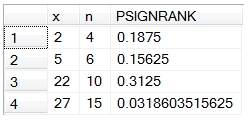# SQL Server Wilcoxon signed rank distribution

PSIGNRANK

Updated: 24 May 2013

Use the scalar function PSIGNRANK to calculate the distribution function of the Wilcoxon Signed Rank statistic.
Syntax
SELECT [wctStatistics].[wct].[PSIGNRANK](
<@X, float,>
,<@N, float,>
,<@Exact, bit,>)
Arguments
@X
the Wilcoxon signed rank statistic. @X is an expression of type float or of a type that can be implicitly converted to float
@N
the number of observations in the sample. @Y is an expression of type float or of a type that can be implicitly converted to float
@Exact
tells the function whether or not to do the exact calculation or to calculate an approximate value using the normal distribution. @Exact is an expression of type bit or of a type that can be implicitly converted to bit

Return Types
Float
Remarks
·         If @N < 0 then an error is returned
·         If @Exact IS NULL and @N > 50 then @Exact = FALSE
·         IF @N > 1023 Then @Exact = FALSE
Examples
SELECT x
,n
,wct.PSIGNRANK(x,n,NULL) as PSIGNRANK
FROM (
SELECT 2,4 UNION ALL
SELECT 5,6 UNION ALL
SELECT 22,10 UNION ALL
SELECT 27,15
)p(x,n)
This produces the following result.### SupportCopyright 2008-2021 Westclintech LLC         Privacy Policy        Terms of Service